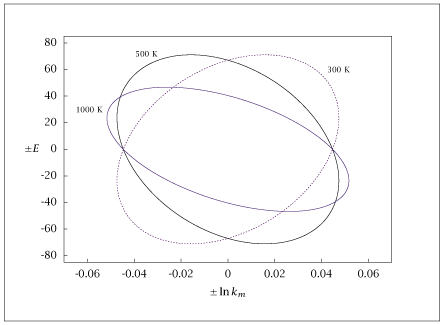## Figure 9.18:

### Effect of next measurement temperature on parameter confidence intervals.## Code for Figure 9.18

### main.m

```
1
2
3
4
5
6
7
8
9
10
11
12
13
14
15
16
17
18
19
20
21
22
23
24
25
26
27
28
29
30
31
32
33
34
35
36
37
38
39
40
41
42
43
44
45
46
47
48
49
50
51
52
53
54
55
56
57
58% Copyright (C) 2001, James B. Rawlings and John G. Ekerdt
%
% This program is free software; you can redistribute it and/or
% modify it under the terms of the GNU General Public License as
% published by the Free Software Foundation; either version 2, or (at
% your option) any later version.
%
% This program is distributed in the hope that it will be useful, but
% WITHOUT ANY WARRANTY; without even the implied warranty of
% MERCHANTABILITY or FITNESS FOR A PARTICULAR PURPOSE.  See the GNU
% General Public License for more details.
%
% You should have received a copy of the GNU General Public License
% along with this program; see the file COPYING.  If not, write to
% the Free Software Foundation, 59 Temple Place - Suite 330, Boston,
% MA 02111-1307, USA.

chisq  = 5.99;  % chi square value for 95% confidence level with 2 parameters
lnk0   = 1;
E      = 100;
Tmin   = 300;
Tmax   = 500;
nTvals = 3;
n0     = 1;
T0     = [ones(n0,1)*Tmin; ones(n0,1)*Tmax];
S      = eye(2);
Trep   = [300, 500, 1000];
meanT  = 1/(0.5*(1/Tmin+1/Tmax));
table  = [];

for i = 1:length(Trep)
Tmeas = [T0; Trep(i)];
ndata = size(Tmeas,1);
Tcenter = -1./Tmeas + 1/meanT;
X = [ones(ndata,1) Tcenter];
measvar = 1e-3;
measstddev = sqrt(measvar);
amat = S*X'*X*S/measvar;
level = chisq;
%
% volume of 95% confidence ellipse and bounding box
%
inva = inv(amat);
intun(i) = sqrt(level*inva(1,1));
slopeun(i) = sqrt(level*inva(2,2));
vol(i) = pi*level/(sqrt(det(amat)));
vbox(i) = 4*level*sqrt(prod(diag(inva)));
[x, y] = ellipse(amat, level, 150);
table = [table x y];
end

save -ascii nextT.dat table;

if (~ strcmp (getenv ('OMIT_PLOTS'), 'true')) % PLOTTING
plot (table(:,1), table(:,2), ...
table(:,3), table(:,4), table(:,5), table(:,6));
% TITLE
end % PLOTTING

```